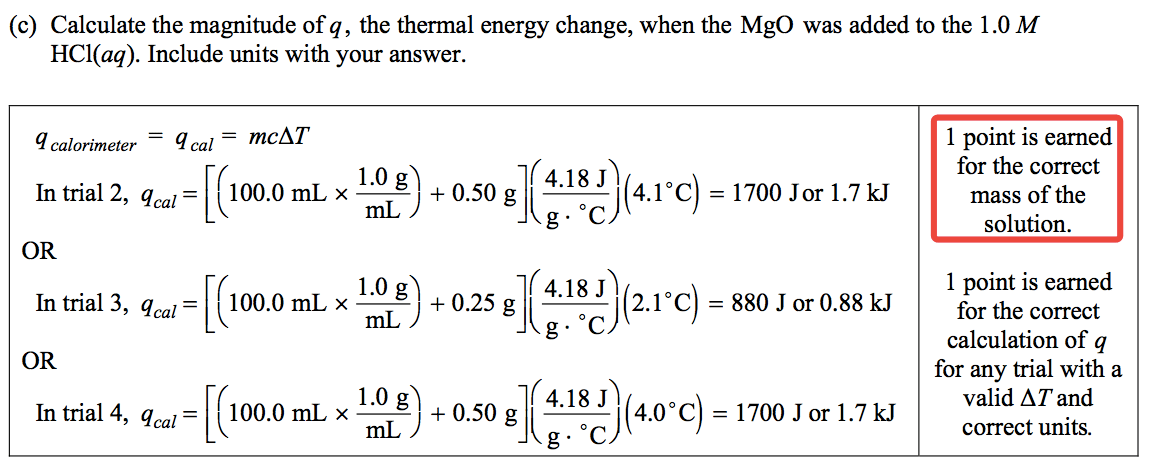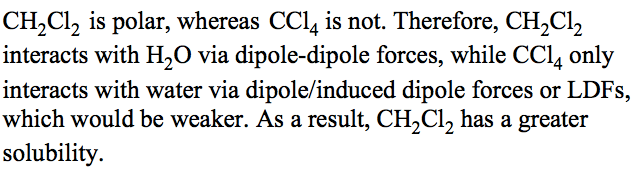# Question 1 (a)

• Write the state of each substance for dissolving equation![The Solubility Expression AaBb(s) aAb+ (aq) + bBa- (aq) Ksp = [Ab+]a [Ba-]b Example:Pb12 (s) Pb2+ + 21- Ksp = [Pb2+1 [I-r *The greater the ksp the more soluble the solid is in 1-120. ](./media/image172.png)

# Question 1 (b)# Question 3 (c)# Question 3 (e)# Question 4 (a)• Write ionic equation if possible

# Question 5 (a)# Question 5 (e)# Question 6 (c)# Question 6 (e)

• hydrogen bonding